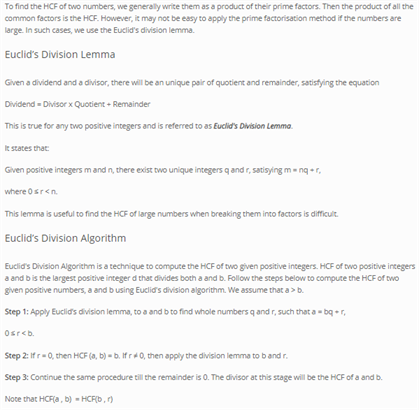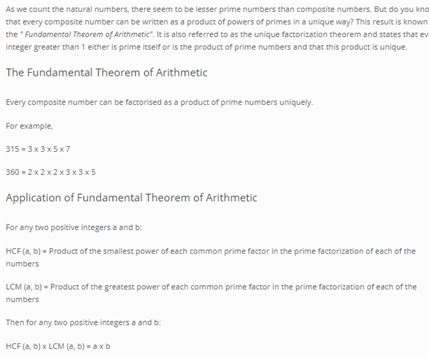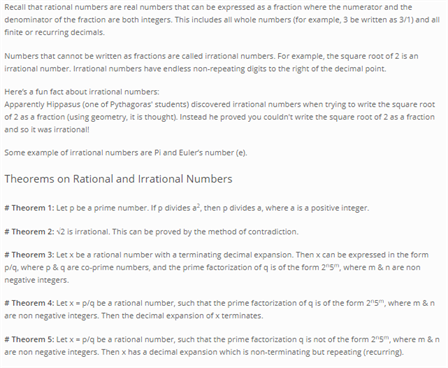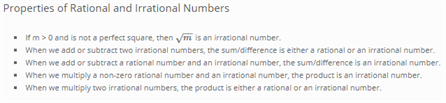# Revision Notes For CBSE Class 10 Maths Chapter 1 Real Numbers

In class 10, students need to have a good knowledge of core math topics and develop effective problem-solving skills in order to succeed in the exams. While there is an extra pressure of appearing for the board exams, students need all the help they can get. Thus, we are providing CBSE revision notes for class 10 maths chapter 1 – Real Numbers here to help students overcome all the challenges in solving math problems. Basically, chapter 1 notes deal with two very important topics – Euclid’s division algorithm and the fundamental theorem of arithmetic which are talked about in properties of positive integers. All the information present in the notes has been carefully put together by our experts and these can be used as a valuable reference tool for understanding all the important chapter topics and questions. Students can also practice and find different ways to solve problems using these notes.

Access free class 10 maths chapter 1 CBSE revision notes PDF below.Access CBSE Class 10 Maths Sample Papers Here.

Access NCERT Class 10 Maths Book Here.